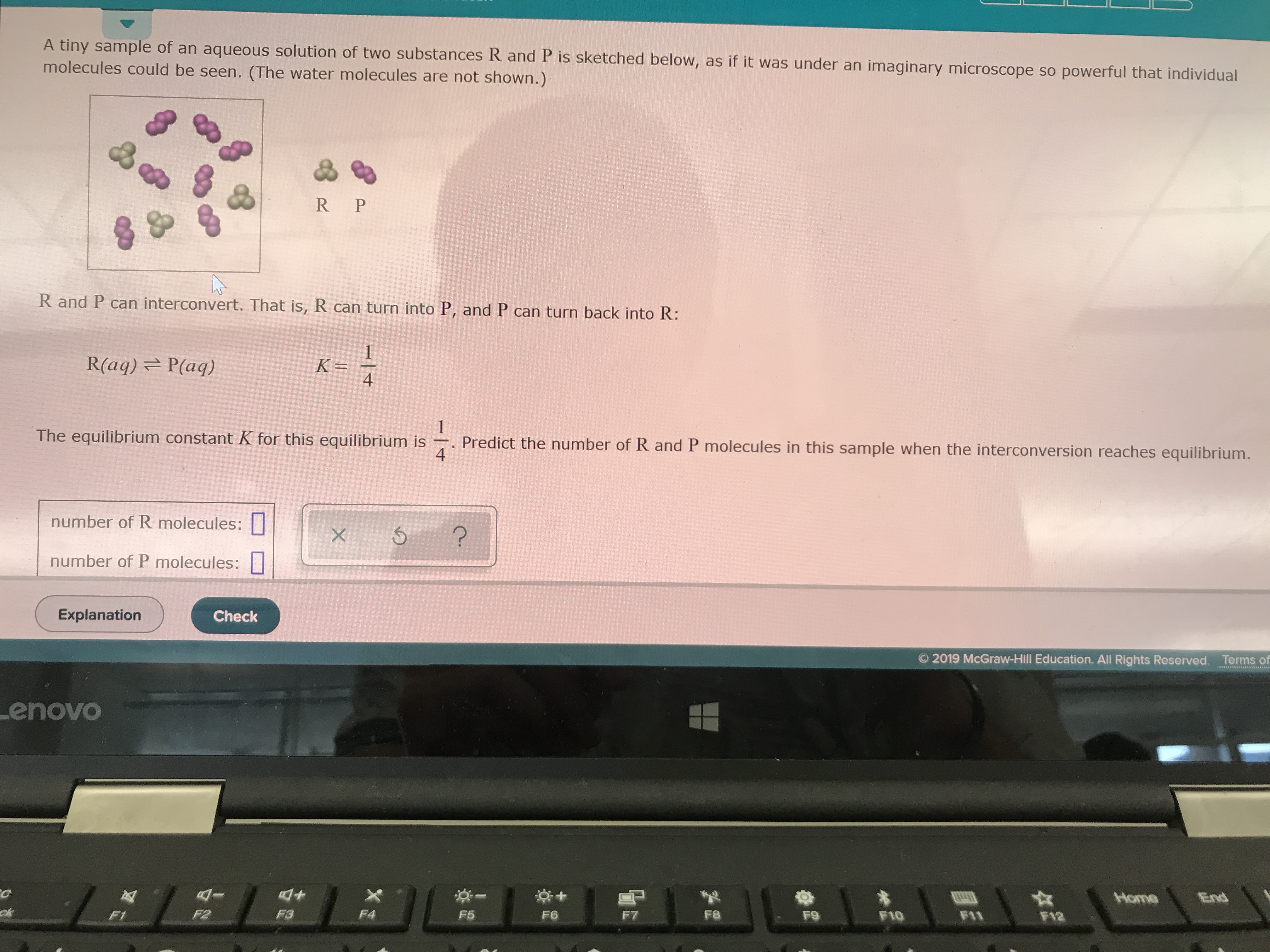# R and P can interconvert. That is, R can turn into P, and P can turn back into R: R(aq) <--> P(aq) K = ¼ The equilibrium constant K for this equilibrium is ¼. Predict the number of R and P molecules in this sample when the interconversion reaches equilibrium